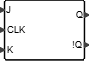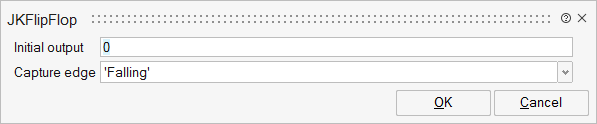# JKFlipFlop

The JK Flip Flop works on a definite portion of the clock cycle (such as the rising edge of the clock). The combination J=0 K=0 holds the state, the combination J=1 K=0 sets the state, the combination J=0 K=1 resets the state, the combination J=1 K=1 toggles the state.FlipFlops

## Description

The JK Flip Flop block works on a definite portion of the clock cycle (such as the rising edge of the clock).

The combination J=0 K=0 holds the state, the combination J=1 K=0 sets the state,the combination J=0 K=1 resets the state,the combination J=1 K=1 toggles the state.

The block is enabled when specified edge is detected, the truth table of inputs and outputs is defined as follows:

J K Q !Q

0 0 Qn-1 !Qn-1

0 1 0 1

1 0 1 0

1 1 !Qn-1 Qn-1

Non-zero is considered true and zero is considered false.

## ParametersNameLabelDescriptionData TypeValid Values

init

Initial output

Output at port Q at initial time

edge

Capture edge

Detect rising or falling edge that enables the block

String

'Rising'
'Falling'

## Ports

NameTypeDescriptionIO TypeNumber

J

explicit

input

2

CLK

explicit

input

2

K

explicit

input

3

Q

explicit

output

1

!Q

explicit

output

2

NameValueDescription

always active

no

direct-feedthrough

yes

zero-crossing

no

mode

no

continuous-time state

no

discrete-time state

no